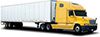# Freight Class Calculator

### How is freight density class calculated?

Let's start with an example shipment. A 40" by 48" pallet is stacked with boxes of products 48" high. The pallet and its contents weighs a total of 425 lbs.

First the volume is calculated by multipled the dimensions together:

40 x 48 x 48 = 92160 cubic inches

Now the volume is converted from cubic inches to feet:

92160 / 1728 = 53.33 cubic feet

To get the density we divide the weight by the volume:

425 / 53.33 = 7.97 pounds per cubic foot

The density is looked up on the table at the left. The density is above 7 but less than 8, so the class is 125.

DensityNMFC Freight Class
5050
3555
3060
2265
1570
1377.5
1285
1092.5
9100
8110
7125
6150
5175
4200
3250
2300
1400
<1500